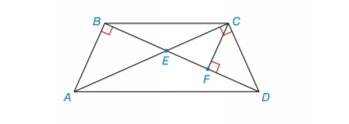Chapter 5.4, Problem 45EElementary Geometry For College St...

7th Edition
Alexander + 2 others
ISBN: 9781337614085

Solutions

Chapter
SectionElementary Geometry For College St...

7th Edition
Alexander + 2 others
ISBN: 9781337614085
Textbook Problem

For quadrilateral A B C D ,   A C ¯ and B D ¯ are diagonals. Also, A B ¯ ⊥ B D ¯ ,   A C ¯   ⊥   C D ¯ , and C F ¯ ⊥ B D ¯ . Give the reason why:a) Δ A B E ∼ Δ C F E b) Δ C F E ∼ Δ D F C c) Δ A B E ∼ Δ D F C [HINT: For part (c), compare the results of (a) and (b)]To determine

a)

The reason for ΔABEΔCFE.

Explanation

Given:

AC¯ and BD¯, AB¯BD¯,AC¯CD¯ and CF¯BD¯ of the quadrilateral.

Definition used:

Property of AA:

“If two angles of one triangle are congruent to two angles of another triangle, then the triangles are similar”

To determine

b)

The reason for ΔCFEΔDFC.

To determine

c)

The reason for ΔABEΔDFC.

Still sussing out bartleby?

Check out a sample textbook solution.

See a sample solution

The Solution to Your Study Problems

Bartleby provides explanations to thousands of textbook problems written by our experts, many with advanced degrees!

Get Started

Find more solutions based on key concepts